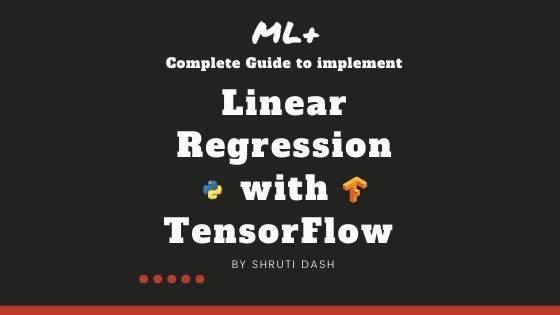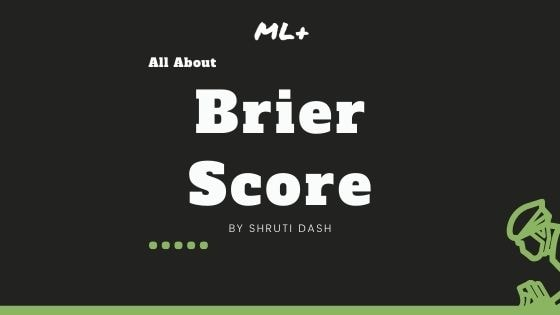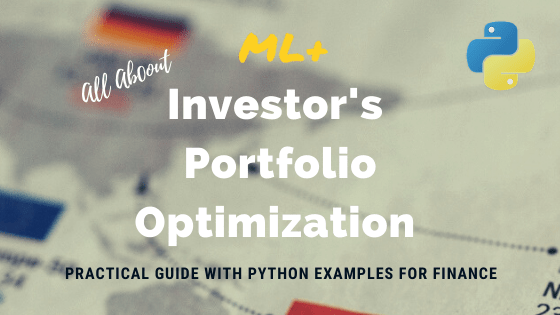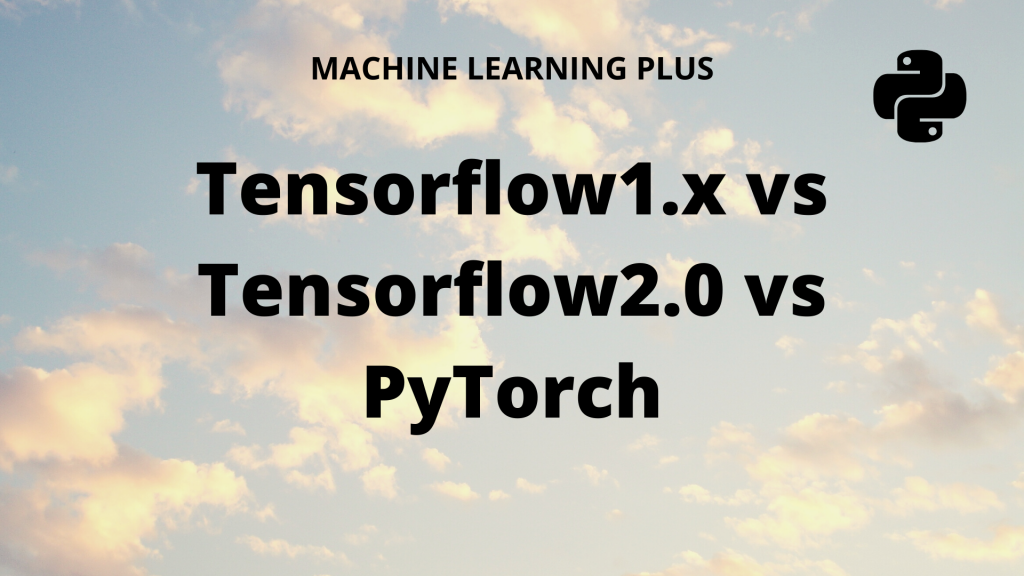# Shruti Dash## How to implement Linear Regression in TensorFlow

Linear Regression is one of the fundamental machine learning algorithms used to predict a continuous variable using one or more explanatory variables (features). In this tutorial, you will learn how to implement a simple linear regression in Tensorflow 2.0 using the Gradient Tape API. Overview In this tutorial, you will understand: Fundamentals of Linear Regression …## Brier Score – How to measure accuracy of probablistic predictions

Brier score is an evaluation metric that is used to check the goodness of a predicted probability score. This is very similar to the mean squared error, but only applied for prediction probability scores, whose values range between 0 and 1. Overview In this tutorial, you will understand: What is Brier score? How is Brier …

## Gradient Boosting – A Concise Introduction from Scratch

Gradient Boosting is a machine learning algorithm, used for both classification and regression problems. It works on the principle that many weak learners (eg: shallow trees) can together make a more accurate predictor. A Concise Introduction to Gradient Boosting. Photo by Zibik How does Gradient Boosting Works? Gradient boosting works by building simpler (weak) prediction …## Portfolio Optimization with Python using Efficient Frontier with Practical Examples

Portfolio optimization in finance is the technique of creating a portfolio of assets, for which your investment has the maximum return and minimum risk. Investor’s Portfolio Optimization using Python with Practical Examples. Photo by Markus In this tutorial you will learn: What is portfolio optimization? What does a portfolio mean? What are assets, returns and …

## How to use tf.function to speed up Python code in Tensorflow

tf.function is a decorator function provided by Tensorflow 2.0 that converts regular python code to a callable Tensorflow graph function, which is usually more performant and python independent. It is used to create portable Tensorflow models.       Introduction Tensorflow released the second version of the library in September 2019. This version, popularly called …## TensorFlow vs PyTorch – A Detailed Comparison

Compare the popular deep learning frameworks: Tensorflow vs Pytorch. We will go into the details behind how TensorFlow 1.x, TensorFlow 2.0 and PyTorch compare against eachother. And how does keras fit in here. Table of Contents: Introduction Tensorflow: 1.x vs 2 Difference between static and dynamic computation graph Keras integration or rather centralization What is …Course Preview

## Machine Learning A-Z™: Hands-On Python & R In Data Science

### Free Sample Videos:#### Machine Learning A-Z™: Hands-On Python & R In Data Science#### Machine Learning A-Z™: Hands-On Python & R In Data Science#### Machine Learning A-Z™: Hands-On Python & R In Data Science#### Machine Learning A-Z™: Hands-On Python & R In Data Science